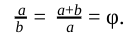top of page
Search

# Introduction to The Golden RatioIntroduction

The golden ratio is found everywhere around us - within the petals of a flower, the spirals of a plant, or in the depths of the night sky. This golden ratio is considered the most pleasing ratio to the human eye, and is commonly found in nature and used in design. Also referred to as the divine proportion or the Greek letter Φ (phi, pronounced fee), this ratio is also the proportion of the side lengths of a golden rectangle. The numerical value of the irrational golden ratio is 1 + 52, which can be approximated to the value 1.618. The golden ratio is very applicable as it is found frequently in our everyday lives and encompasses many intriguing patterns and properties.The Golden Ratio in History

In history, the golden ratio can be found in many architectural structures, books, and also famous paintings. The earliest example of the golden ratio can be observed in the Pyramids of Giza, where the height to base ratio is approximately 1.618. Although the first example of the golden ratio was found in the Pyramids, many believe Phidias had first discovered this mysterious golden ratio, and that phi was named after him. A sculptor, painter, and architect, Phidias had directed the construction of the Parthenon, a temple dedicated to the Greek goddess Athena in 447 BC. There are various golden rectangles found throughout the Parthenon, including the ratio of its height to width.Finding the Golden Ratio

One method to find the golden ratio is using the golden rectangle- a rectangle with side lengths in the golden ratio, then creating an equation and solving it using algebra. Here, note that a golden rectangle has an exceptional property that, if broken into a square and a rectangle, the ratio of the square’s side length to the shorter part of the length will be equal to the ratio of the whole length to the width.This can become an algebraic equation:This fraction can be simplified to 1.